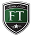# Discounted Cash Flow Valuations

The following video is borrowed from our BusinessTraining.com platform and was originally recorded for our financial modeling training program.  There are many ways to value a company, each with its own merit and discounted cash flow valuation is one such method.  In the following video, you see how to conduct a discounted cash flow valuation.

Video Transcript/SummaryThe strategies and tips provided within this video module include:

1. Discounted Cash Flow (DCF) is one valuation method which discounts the expected future cash flows. DCF works of the concept; what will someone pay today for a future set of cashflows. A discount factor needs to be used to factor in risks. The present value of future cash flows is known as Enterprise Value.
2. Step 1 – Estimate EBIT, calculate taxes due and subtract taxes from EBIT, add back depreciation and amortisation and subtract routine maintenance capital expenses to determine the Free Cash Flow figure for each period, which is the figure you will use for discounting.
3. Models typically project out to 5 years. In order to  project further out, a terminal value needs to be calculated. One approach might be to discount the 5th year FCF projection to eternity. Alternatively, an exit multiple based on comparable can be used.
4. Now the FCF and terminal values need to be discounted back to present values. This discounted figure is the Weighted Average Cost of Capital (WACC), which is based on the equity and debt of the company being valued.
5. Cost of Equity (Re) = Risk-free rate (Rf) + (Beta * Risk Premium). Rf is typically what you can get from a 10 year treasury, Beta measures the risk of a company relative to the broader market and Risk premium is company specific and includes factors such as revenue, liquidity, etc.
6. Cost of debt (Rd) = Weighted average interest rate on borrowings. Equity % =  equity Capital / Total Capital and similarly, Debt % = Debt Capital / Total Capital.
7. Projected cash flows are at the end of the year but realistically, the business generates cash flow throughout the year. Therefore, modellers generally prefer to use Mid-Period discounting but this is not the case without the terminal value calculation, just the individual cash flow projections. Once these are all added up, the Enterprise Value (EV) is reached.
8. EV + Cash – Debt = Equity Value, where Equity Value is a proxy for the market capitalisation of the company. Equity Value / Total Shares Outstanding = DCF price per share.

Learning a variety of valuation methods is essential to succeeding as a financial professional.  Discounted cash flow valuation is a useful way to ascertain the value of a company.Your friends here at https://investmentcertifications.com

Join 11,626 Investment Certification Experts!
• Please choose what program you have an interest in learning more about.

CALL OR MESSAGE FOR SUPPORT

## Contact

328 Crandon Blvd
Suite 225
Key Biscayne, FL 33149

E-Mail

Phone
+1 (305) 503-9050